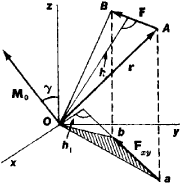# Moment of Force

Also found in: Dictionary, Thesaurus, Medical, Legal, Acronyms.

## moment of force

[′mō·mənt əv ′fȯrs]
(mechanics)
McGraw-Hill Dictionary of Scientific & Technical Terms, 6E, Copyright © 2003 by The McGraw-Hill Companies, Inc.
The following article is from The Great Soviet Encyclopedia (1979). It might be outdated or ideologically biased.

## Moment of Force

a quantity that characterizes the rotational effect of a force acting on a rigid body; the moment of a force is a fundamental concept of mechanics. A distinction is made between the moment of a force about a center (a point) and about an axis.

The moment of a force about a center O is a vector quantity. The magnitude of the moment is MO = Fh, where F is the force and h is the arm, that is, the length of the perpendicular drawn from O to the line of action of the force (see Figure 1); the vector MO is perpendicular to the plane that contains the center O and the force F and is pointed in a direction such that the rotation caused by the force appears counterclockwise (in a right-handed coordinate system). The moment of a force is expressed as a vector product, by the equation MO = [rF], where r is the radius vector drawn from O to the point of applicationFigure 1

of the force. The dimensions of the moment of a force are L2MT2, and the units of measurement are newton ⋅ meters (n ⋅ m), dyne ⋅ cm (1 N ⋅ m = 107 dyne ⋅ cm), or kilogram-force ⋅ meters.

The moment of a force about an axis is a scalar quantity equal to the projection, on this axis, of the moment of the force about any point O of the axis or equal to the numerical value of the moment of the projection Fxy of the force F on the xy-plane, which is perpendicular to the z-axis, taken with respect to the point of intersection of the axis with the plane. In other words,

MZ = M0 cos γ = ± Fxyh1

The plus sign in this expression is selected when the rotation caused by the force F is counterclockwise when viewed from the positive end of the z-axis (also in a right-handed system). The moments of a force about the x-, y-, and z-axes can also be calculated from the formulas

Mx = YFzZFyMy = zFxxFz

where Fx, Fy, and Fz are the projections of the force F on the axes; x,y, and z are the coordinates of the point A of application of the force.

If a system of forces has a resultant, then the moment of the system is calculated according to Varignon’s theorem.

S. M. TARG